# Test Prep Plan - Take a practice test

Take this practice test to check your existing knowledge of the course material. We'll review your answers and create a Test Prep Plan for you based on your results.
How Test Prep Plans work
1
2Based on your results, we'll create a customized Test Prep Plan just for you!
3Study smarter
Study more effectively: skip concepts you already know and focus on what you still need to learn.

# NMTA Math: Graphing Derivatives Chapter Exam

Exam Instructions:

Choose your answers to the questions and click 'Next' to see the next set of questions. You can skip questions if you would like and come back to them later with the yellow "Go To First Skipped Question" button. When you have completed the practice exam, a green submit button will appear. Click it to see your results. Good luck!

### Page 1

#### Question 1 1. What is the slope of a line normal to f(x)?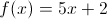#### Question 2 2. The graph shown is an example of a function that: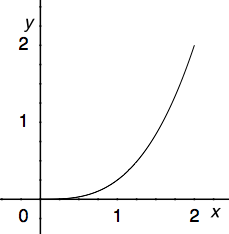#### Question 3 3. The derivative of the following graph is _____ at point D.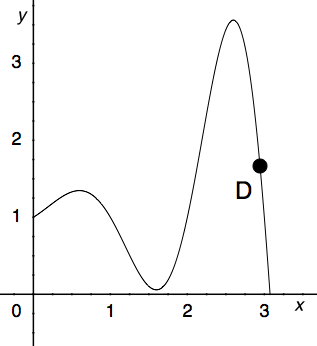#### Question 4 4. What is the slope of the tangent to r(s) at s = 0?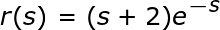### Page 2

#### Question 6 6. Consider the function h(x) below. At x = 1 is this function concave up, concave down, or an inflection point?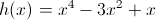#### Question 7 7. What is the slope of the line normal to f(x) at x=1?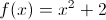#### Question 8 8. Which of the following is a local minimum?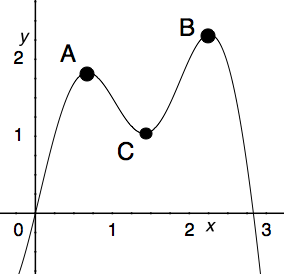#### Question 9 9. What is the equation of the line tangent to f(x) at the point x=0?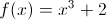### Page 3

#### Question 11 11. Consider the function g(x) below. At x = 0 is this function concave up, concave down, or an inflection point?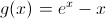#### Question 12 12. Over the region shown, the global minimum is at the point#### Question 13 13. What is the global minimum for the function y=f(x) below between x=0 and x=2?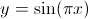#### Question 14 14. The derivative of the following graph is _____ at point C.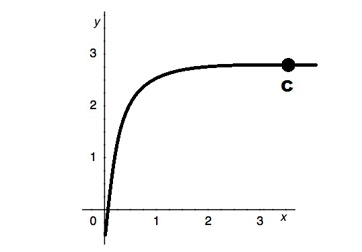#### Question 15 15. The graph shown is an example of a function that: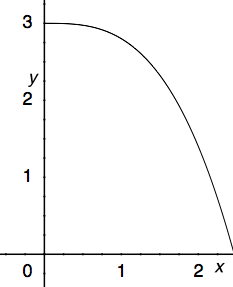### Page 4

#### Question 16 16. Consider the function f(x) below. At x = 1 is this function concave up, concave down, or an inflection point?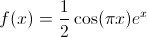#### Question 17 17. Which could be the graph of the derivative of the function below?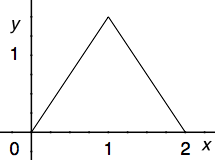#### Question 18 18. Between x=1 and x=3, the following function _____.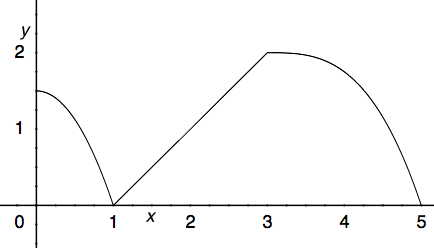#### Question 19 19. Where is the global maximum for the function y=f(x) below between x=0 and x=2?### Page 5

#### Question 21 21. Which of the following BEST describes point C?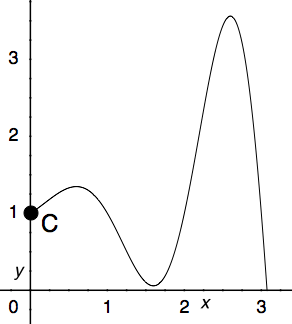#### Question 23 23. What is the equation of the line normal to f(x) at the point x=1?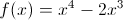#### Question 25 25. What is value of y at the finite local maximum for the function y=f(x) below?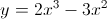### Page 6

#### Question 26 26. Consider the function f(x) below. At x = 2 is this function concave up, concave down, or an inflection point?#### Question 27 27. Which of the following BEST describes point B?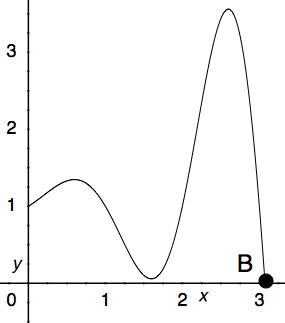#### Question 28 28. The graph shown is an example of a function that: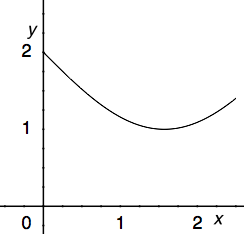#### Question 29 29. Over the region shown, point A is known as _____.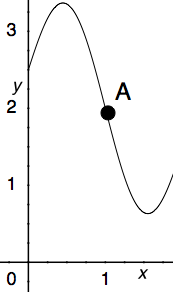#### Question 30 30. What is the global minimum for the function y=f(x) below between x=0 and x=2?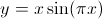#### NMTA Math: Graphing Derivatives Chapter Exam Instructions

Choose your answers to the questions and click 'Next' to see the next set of questions. You can skip questions if you would like and come back to them later with the yellow "Go To First Skipped Question" button. When you have completed the practice exam, a green submit button will appear. Click it to see your results. Good luck!

Support